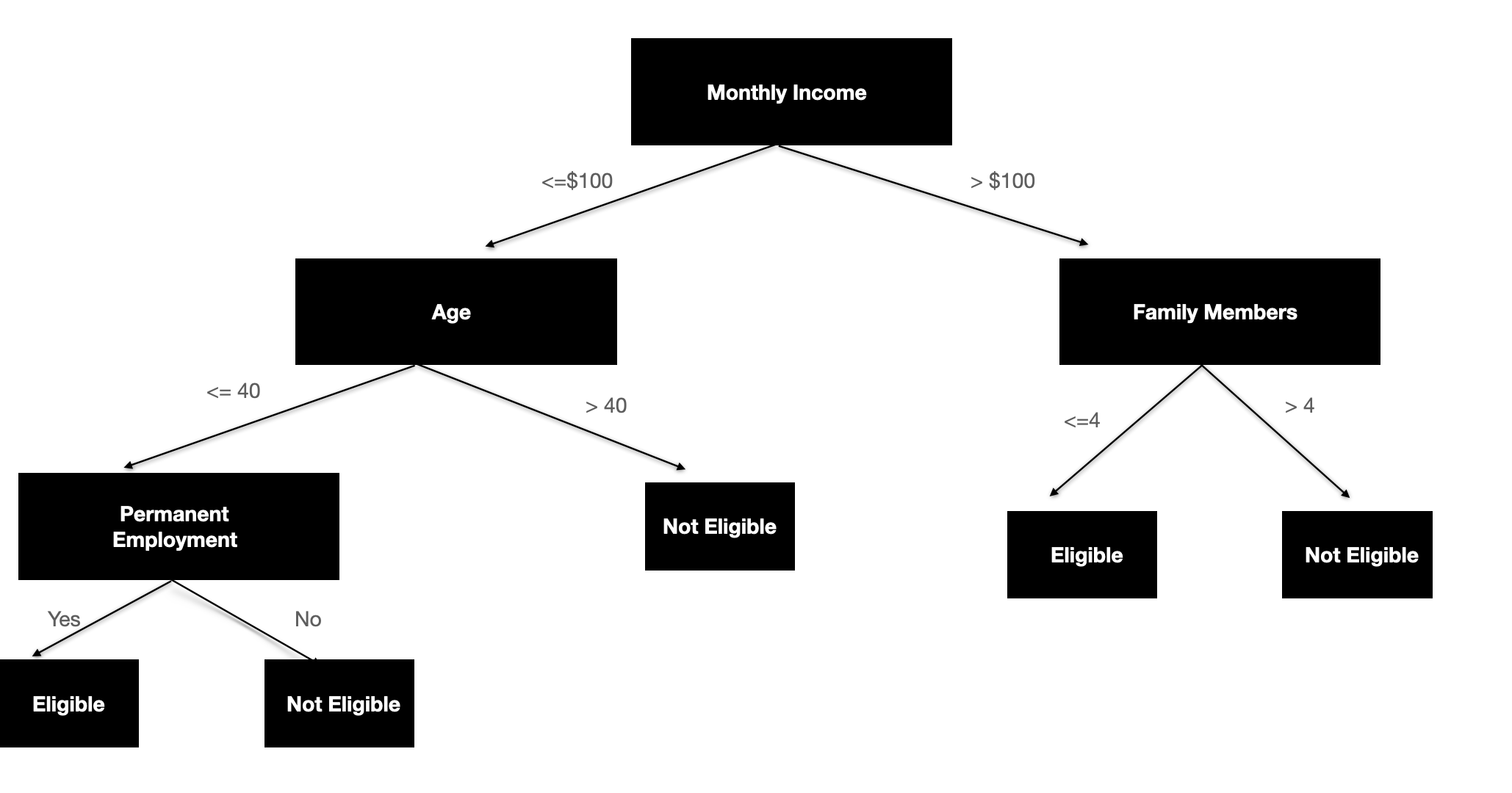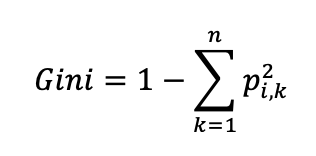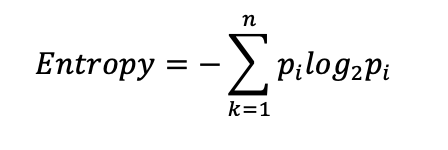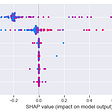# Decision Trees Which Never Gets Old

If you are working with clients and have worked with many , there is a high chance that at least once you have met a client who is interested in how you are providing him the solution, what’s the concept behind it and struggles to believe through and through in any unexplainable models even though there is a high chance of obtaining higher accuracy. This is the nature of humans and would be sometimes understandable if they are not willing to accept an unexplainable solution to be implemented in their own business.

Let’s consider the below 2 scenarios where a business wants a solution which continuously increase a parameter called Z given A, B, C inputs.

1. Scenario 01:
Underlying technique of the model is A+B — C= Z.

- This is much easily interpreted and business will easily understand we have to either increase A or B or decrease C to maximise Z.
2. Scenario 02:
Underlying technique of the model is a black box .
- For example a mathematical calculations through layers. It will have hundreds of layers and at each layer a calculation will happen to inputs and at the end it will provide a very accurate prediction.

This will make no sense to the business team and they might not have the confidence to use the model to generate the output however much the accurate the end result is.

Therefore, in some situations getting the business team onboard with an interpretable model becomes more important than providing a very accurate output with a complex non interpretable algorithm.

Decision tree is one such simple fundamental modelling technique which is very easy to understand by any non technical person. By reading out the nodes, how the prediction will be made can be easily sorted out. This might be the reason even over the past decades it has never gone out of style.

# What is a decision tree?fig 2: Image from Unplash by Alla Hetman, fig3: Image by Author

Decision tree is basically an upside down tree like structure starting with a root node with a set of rules as branches to arrive at the decision at the leaf node.

Root : Root Node with data representing the whole population

Branches : Represent the rules which splits the data of the parent node

Internal Nodes / Leaf Nodes : The other decision points apart from the root node. If the dataset of the node is split again, that node is called an internal node or if it’s at the end of the decision tree branch which carries the decision point its called a leaf node.

## How the decision tree is built?

When a decision is made in normal circumstances more than one attribute takes part in the decision making process, however all the attributes might not have the same relevance or the importance towards the final decision. Decision tree tries to find this importance or relevance by looking at the training data.

To decide this, there are several splitting criterions used across multiple algorithms. Most of them try to get to homogeneous leaf nodes.When decision tree is built in this manner, it places the node which splits the data into the most homogeneous groups at the root node and while traversing down to the leaf nodes it chooses the nodes and rules to split at each step so that each set of groups has more homogeneity leading to a better classification. A common term used here is the impurity of nodes. More a node is homogeneous lesser its impurity would be.

Below are some popular splitting criterions,

• Gini Index
Gini Index indicates the probability of an instance being classified wrongly when chosen randomly. It measures the mix of the classes of the target variable by calculating their probability distributions.where k is the number of classes varying from 1 to n and p is the probability of the instance being classified to a particular class.

If all elements belong to the same class
Gini = 1 — (¹² + ⁰² + ⁰² +….) = 0 indicating the node being very pure.
If the classes are randomly distributed Gini would be higher and when the classes are equally distributed it would be 0.5.
Therefore when splitting we try to choose the node which provides the least Gini impurity index.

• Entropy
Entropy measures the lack of predictability of a branch, in other words the uncertainty of a distribution. When building a decision tree, it aims to reduce the level of entropy at each split, thus the node which provides the least entropy would be chosen.where k is the number of classes varying from 1 to n and p is the probability of the instance being classified to a particular class.
• Information Gain (ID3)
Information Gain measures how much information a feature gives about a class. If a node gives more information then that node is selected to be the splitting node. Higher information gain could be achieved by having a lesser randomness among the classes within a node.
This concept is also related to entropy as information gain is actually a measure of decrease in entropy after splitting the dataset. If there is higher decrease in entropy leading to more predictability , there is a greater information gain.
• Chi square test
As the splitting node this chooses the variable which has the most significant relationship with the target variable. When there is no such significance, non significant categories of predictors are merged when building the decision tree.
• F test
This calculates the statistical difference of means of two populations. If two populations are not significantly different they are merged together when building the decision tree.
• Least square deviation
This tries to minimise the sum of squared distances between observed vs predicted values (i.e residuals). Node which provides the least value for residuals is chosen as the splitting node.

## What to look out for?

As discussed, building a decision tree is easy and it is one of the most interpretable models which exists. So what’s the catch here.

• Over fitting the data
When a decision tree is built deeper and deeper it tries to isolate even a single data point if the split will be purer. As we can see in the above image, the star in the right hand side is most probably an outlier. If the decision tree is trained like this when predicting it’ll be predicted as a star when a new data point falls into that area where it is probably not a star. This is called overfitting. This happens because when decision tree goes on splitting data to smaller sample sizes, strict rules will be generated for the sparse data which perfectly fits the training data but not to any other general data set. So there is this intrinsic nature of the decision tree to be prone to overfit the data, if necessary steps are not taken to overcome this.
• Instability which comes with the greedy nature
When a decision tree is built it looks at the immediate optimal solution which gives the optimal next split. This is called a greedy nature of an algorithm. So the overall solution might not be the most optimal solution and when totally new data is introduced there is a possibility for the splitting nodes to be changed with the information of the new data and by propagating it down, the tree decision tree could change.
• Problem with unbalanced data
This is one of the most common problem seen across many machine learning algorithms. If necessary steps are not taken before training the model decision tree will have a bias towards the dominating class.

## How to find the silver lining amidst the dark cloud?

In one hand the decision tree is one of the best interpretable models but on the other hand accuracy of the model will be forsaken if the model is faced with the above issues. However, there are multiple techniques to minimise these pitfalls.

• Assigning a maximum depth
One method is to specify a maximum depth to the algorithm. For example in the above scenario if the maximum depth is assigned as 2 the split which isolates the one star would not happen.
• Assigning minimum instances per node
Another method is to assign minimum number of data points to be there after a node is split. In the above example if it is specified as 3 again the star we are talking about wont be isolated. When it is specified the model does not try to generate rules from less number of data points.
• Assigning a ratio between majority and minority classes
This is another method to reduce the overfitting . For example we can stop the splitting when 90% of the points belongs to one class
• Use pruning techniques
As the name suggests this cuts off the branches and tries to get a well off tree by removing redundant splits. This could be done in either when building up the tree called as pre pruning or early stopping or after fitting the tree by cutting back the redundant splits called as post pruning. The criteria to find out redundant splits differs. In some methods it checks information gain, cross validated errors etc and tries to get a smaller tree which is not fitted perfectly only on the training set.
• Hyper parameter tuning
Although the decision tree does not need to have a proper feature selection to be done first as the tree tends to pick up the best parameter which splits the data, it is always better to iterate the model with subsets of features alongside with the techniques such as hyper parameter tuning and cross validation.
• Balance the dataset
When there is unequal distribution of classes in the dataset we say the dataset is unbalanced. Problem here is, in classification problems, models become more bias towards the majority class as those set of data is more prominent. For example in a dataset about cancer test from the 100 sample if 98 are benign the model might predict all as benign and still have a higher accuracy. Therefore, it is always best to use techniques such as under sampling, oversampling etc. and treat the dataset before modelling.
• Experimentation with domain knowledge
Although mentioned as the last point this is one of the most important thing to do. It is always better to check whether the model rules makes business sense without only depending on any evaluation metrics, specially if the dataset is not too large. When decision trees splits change with new data or when class imbalance exists this experimentation becomes a very handy tool.

Like above, if we are cautious when building the model most of these limitations could be minimised and would be able to provide a well balanced model to satisfy the business needs.

It would be incomplete if we do not talk about the bright side of the decision trees too, apart from the interpretability. Below are some of those.

• Being a non parametric model no assumptions has to be made about the underlying dataset
• No scaling or normalisation is required as when the split is happening single feature is at focus
• No feature selection is required as the top nodes in the decision tree will be naturally the most important feature

## Decision Tree Algorithms

There are several popular algorithms to build decision trees.

They primarily differ by,

• choice of the splitting criterion
• Ability to fit regression or classification decision trees
• Handling missing values or imbalance data
• Techniques handling overfitting

Some algorithms are listed below.

1. ID3 (Iterative Dichotomiser)
- Can be used for both binary and multiple classifications
- Uses Shannon Entropy to pick features with the greatest information gain as nodes
- Splitting criteria is the split which has the largest information gain (or the smallest entropy).
- Splitting will recursively happen until the node is homogeneous.
- This uses a greedy search and it selects a node using the information gain criterion, and then never explores the possibility of alternate choices.
- This doesn’t handle missing values or numerical data
2. C4.5
- This is the successor of ID3 algorithm
- Splitting criteria is the information gain ratio
- This can handle both continuous and discrete attributes.
For continuous variables, it creates a threshold and then splits the list into those whose attribute value is above the threshold and those that are less than or equal to it.
- Can use missing values and are simply not used in calculations of gain and entropy.
- Weights can be applied for the features that comprise the data
- Allow post pruning
3. CART (Classification and Regression Models)
- This algorithm generates both classification and regression trees
- Used for binary classification
- When selecting feature to split gini index is used in case of classification tree and least square deviation is used in regression trees
4. CHAID ( Chi squared Automatic Interaction Detetction)
- This produces multiple splits
- used for both regression and classification tasks
- no replacement of missing values
- no pruning ability
- use chi square independence test to determine the best split in classification trees and F test in regression trees

Apart from these algorithms like C5.0, CHAID, MARS etc. also exists.

## Final Thoughts

Decision trees have its own faults like overfitting, sub optimization etc, but when it comes to explainability and using it for simpler problems with simpler data with necessary steps taken against the pitfalls, decision trees will be a really valuable option.

In the war between the interpretability and accuracy, if there is a chance to make the accuracy wins amidst other models decision trees have its own extensions in bagging and boosting like random forests where the probability of over fitting and instability due to variance is reduced leading to more robust solutions.

--

--

--

## More from MLearning.ai

Data Scientists must think like an artist when finding a solution when creating a piece of code. ⚪️ Artists enjoy working on interesting problems, even if there is no obvious answer ⚪️ linktr.ee/mlearning 🔵 Follow to join our 18K+ Unique DAILY Readers 🟠

## The Diversity of Opportunity in Data Science: Company Information Sessions with PwC, Philadelphia…## Topic Modeling of War articles and Other articles -2-## Movies in Words## Big data checklist: all you need to make better data-driven decisions## Machine Learning Model Explanation## Data Transformation and other valuable things.Data Scientist

## Sports Person Classification## The shape of data to come## The Perceptron Algorithm## The Math Behind the K-means and Hierarchical Clustering Algorithm!